Search by Topic

Resources tagged with Maths Supporting SET similar to Over-booking:

Filter by: Content type:
Age range:
Challenge level:

There are 89 results

Broad Topics > Applications > Maths Supporting SETOver-booking

Age 16 to 18 Challenge Level:

The probability that a passenger books a flight and does not turn up is 0.05. For an aeroplane with 400 seats how many tickets can be sold so that only 1% of flights are over-booked?Pdf Stories

Age 16 to 18 Challenge Level:

Invent scenarios which would give rise to these probability density functions.The Wrong Stats

Age 16 to 18 Challenge Level:

Why MUST these statistical statements probably be at least a little bit wrong?Population Dynamics Collection

Age 16 to 18 Challenge Level:

This is our collection of tasks on the mathematical theme of 'Population Dynamics' for advanced students and those interested in mathematical modelling.Real-life Equations

Age 16 to 18 Challenge Level:

Here are several equations from real life. Can you work out which measurements are possible from each equation?Stirling Work

Age 16 to 18 Challenge Level:

See how enormously large quantities can cancel out to give a good approximation to the factorial function.Global Warming

Age 14 to 16 Challenge Level:

How much energy has gone into warming the planet?Bessel's Equation

Age 16 to 18 Challenge Level:

Get further into power series using the fascinating Bessel's equation.Approximately Certain

Age 14 to 18 Challenge Level:

Estimate these curious quantities sufficiently accurately that you can rank them in order of sizeAim High

Age 16 to 18 Challenge Level:

How do you choose your planting levels to minimise the total loss at harvest time?Whose Line Graph Is it Anyway?

Age 16 to 18 Challenge Level:

Which line graph, equations and physical processes go together?Scale Invariance

Age 16 to 18 Challenge Level:

By exploring the concept of scale invariance, find the probability that a random piece of real data begins with a 1.Transformations for 10

Age 16 to 18 Challenge Level:

Explore the properties of matrix transformations with these 10 stimulating questions.Guessing the Graph

Age 14 to 16 Challenge Level:

Can you suggest a curve to fit some experimental data? Can you work out where the data might have come from?Big and Small Numbers in the Living World

Age 11 to 16 Challenge Level:

Work with numbers big and small to estimate and calculate various quantities in biological contexts.Time to Evolve 2

Age 16 to 18 Challenge Level:

How is the length of time between the birth of an animal and the birth of its great great ... great grandparent distributed?Big and Small Numbers in the Physical World

Age 14 to 16 Challenge Level:

Work with numbers big and small to estimate and calculate various quantities in physical contexts.Building Approximations for Sin(x)

Age 16 to 18 Challenge Level:

Build up the concept of the Taylor seriesDangerous Driver?

Age 16 to 18 Challenge Level:

Was it possible that this dangerous driving penalty was issued in error?Designing Table Mats

Age 11 to 16 Challenge Level:

Formulate and investigate a simple mathematical model for the design of a table mat.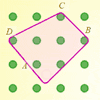Elastic Maths

Age 14 to 18

How do you write a computer program that creates the illusion of stretching elastic bands between pegs of a Geoboard? The answer contains some surprising mathematics.Big and Small Numbers in Physics

Age 14 to 16 Challenge Level:

Work out the numerical values for these physical quantities.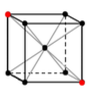Crystal Symmetry

Age 16 to 18 Challenge Level:

Use vectors and matrices to explore the symmetries of crystals.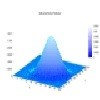Pdf Matcher

Age 16 to 18 Challenge Level:

Which pdfs match the curves?Constantly Changing

Age 14 to 16 Challenge Level:

Many physical constants are only known to a certain accuracy. Explore the numerical error bounds in the mass of water and its constituents.Vector Walk

Age 14 to 18 Challenge Level:

Starting with two basic vector steps, which destinations can you reach on a vector walk?Fix Me or Crush Me

Age 16 to 18 Challenge Level:

Can you make matrices which will fix one lucky vector and crush another to zero?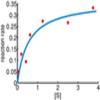Curve Fitter 2

Age 16 to 18 Challenge Level:

Can you construct a cubic equation with a certain distance between its turning points?Scientific Curves

Age 16 to 18 Challenge Level:

Can you sketch these difficult curves, which have uses in mathematical modelling?Polygon Walk

Age 16 to 18 Challenge Level:

Go on a vector walk and determine which points on the walk are closest to the origin.Square Pair

Age 16 to 18 Challenge Level:

Explore the shape of a square after it is transformed by the action of a matrix.Matrix Meaning

Age 16 to 18 Challenge Level:

Explore the meaning behind the algebra and geometry of matrices with these 10 individual problems.Nine Eigen

Age 16 to 18 Challenge Level:

Explore how matrices can fix vectors and vector directions.Big and Small Numbers in Chemistry

Age 14 to 16 Challenge Level:

Get some practice using big and small numbers in chemistry.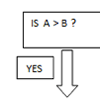Mystery Procedure

Age 14 to 16 Challenge Level:

Can you work out what this procedure is doing?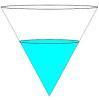Fill Me up Too

Age 14 to 16 Challenge Level:

In Fill Me Up we invited you to sketch graphs as vessels are filled with water. Can you work out the equations of the graphs?Investigating Epidemics

Age 11 to 16 Challenge Level:

Simple models which help us to investigate how epidemics grow and die out.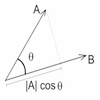Cross with the Scalar Product

Age 16 to 18 Challenge Level:

Explore the meaning of the scalar and vector cross products and see how the two are related.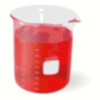Brimful 2

Age 16 to 18 Challenge Level:

Which of these infinitely deep vessels will eventually full up?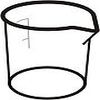Brimful

Age 16 to 18 Challenge Level:

Can you find the volumes of the mathematical vessels?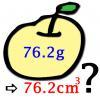More or Less?

Age 14 to 16 Challenge Level:

Are these estimates of physical quantities accurate?Electric Kettle

Age 14 to 16 Challenge Level:

Explore the relationship between resistance and temperatureQuorum-sensing

Age 16 to 18 Short Challenge Level:

This problem explores the biology behind Rudolph's glowing red nose.Biology Measurement Challenge

Age 14 to 16 Challenge Level:

Analyse these beautiful biological images and attempt to rank them in size order.Far Horizon

Age 14 to 16 Challenge Level:

An observer is on top of a lighthouse. How far from the foot of the lighthouse is the horizon that the observer can see?Air Routes

Age 16 to 18 Challenge Level:

Find the distance of the shortest air route at an altitude of 6000 metres between London and Cape Town given the latitudes and longitudes. A simple application of scalar products of vectors.Production Equation

Age 16 to 18 Challenge Level:

Each week a company produces X units and sells p per cent of its stock. How should the company plan its warehouse space?Differential Equation Matcher

Age 16 to 18 Challenge Level:

Match the descriptions of physical processes to these differential equations.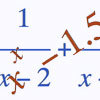Root Hunter

Age 16 to 18 Challenge Level:

In this short problem, try to find the location of the roots of some unusual functions by finding where they change sign.Perspective Drawing

Age 11 to 16 Challenge Level:

Explore the properties of perspective drawing.